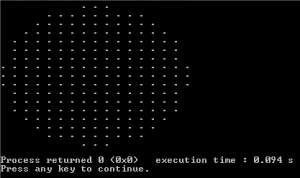# Draw a circle without floating point arithmetic

• Last Updated : 01 Jun, 2022

Given a radius of a circle, draw the circle without using floating point arithmetic.
Following program uses a simple concept. Let the radius of the circle be r. Consider a square of size (2r+1)*(2r+1) around the circle to be drawn. Now walk through every point inside the square. For every  point (x,y), if (x, y) lies inside the circle (or x^2+ y^2 < r^2), then print it, otherwise print space.

## C++

 `// C++ program to draw a circle without``// floating point arithmetic``#include ` `void` `drawCircle(``int` `r)``{``    ``// Consider a rectangle of size N*N``    ``int` `N = 2*r+1;` `    ``int` `x, y;  ``// Coordinates inside the rectangle` `    ``// Draw a square of size N*N.``    ``for` `(``int` `i = 0; i < N; i++)``    ``{``        ``for` `(``int` `j = 0; j < N; j++)``        ``{``            ``// Start from the left most corner point``            ``x = i-r;``            ``y = j-r;` `            ``// If this point is inside the circle, print it``            ``if` `(x*x + y*y <= r*r+1 )``                ``printf``(``"."``);``            ``else` `// If outside the circle, print space``                ``printf``(``" "``);``            ``printf``(``" "``);``        ``}``        ``printf``(``"\n"``);``    ``}``}` `// Driver Program to test above function``int`  `main()``{``    ``drawCircle(8);``    ``return` `0;``}`

## Java

 `// Java program to draw a circle without``// floating point arithmetic` `import` `java.io.*;` `class` `GFG {``static` `void` `drawCircle(``int` `r)``{``    ``// Consider a rectangle of size N*N``    ``int` `N = (``2``*r+``1``);` `    ``int` `x, y; ``// Coordinates inside the rectangle` `    ``// Draw a square of size N*N.``    ``for` `(``int` `i = ``0``; i < N; i++)``    ``{``        ``for` `(``int` `j = ``0``; j < N; j++)``        ``{``            ``// Start from the left most corner point``            ``x = i-r;``            ``y = j-r;` `            ``// If this point is inside the circle, print it``            ``if` `(x*x + y*y <= r*r+``1` `)``                    ``System.out.print(``"."``);``            ``else` `// If outside the circle, print space``                    ``System.out.print(``" "``);``                ``System.out.print(``" "``);``        ``}``            ``System.out.println();``    ``}``}` `// Driver Program to test above function``    ``public` `static` `void` `main (String[] args) {``        ``drawCircle(``8``);``    ``}``//This code is contributed by ajit.``}`

## Python3

 `# Python3 program to draw a circle without``# floating point arithmetic``def` `drawCircle(r) :``    ` `    ``# Consider a rectangle of size N*N ``    ``N ``=` `2``*``r ``+` `1``  ` `    ``# Draw a square of size N*N. ``    ``for` `i ``in` `range``(N) :    ``        ``for` `j ``in` `range``(N) :``        ` `            ``# Start from the left most corner point ``            ``x ``=` `i ``-` `r ``            ``y ``=` `j ``-` `r``  ` `            ``# If this point is inside the circle, print it ``            ``if` `(x ``*` `x ``+` `y ``*` `y <``=` `r ``*` `r ``+` `1` `) :``                ``print``(``"."``, end ``=` `"")``            ``else` `:``# If outside the circle, print space ``                ``print``(``" "``, end ``=` `"") ``            ``print``(``" "``, end ``=` `"")``            ` `        ``print``()``            ` `drawCircle(``8``)` `# This code is contributed by divyeshrabadiya07.`

## C#

 `// C#  program to draw a circle without``// floating point arithmetic` `using` `System;` `public` `class` `GFG{``    ``static` `void` `drawCircle(``int` `r)``{``    ``// Consider a rectangle of size N*N``    ``int` `N = (2*r+1);` `    ``int` `x, y; ``// Coordinates inside the rectangle` `    ``// Draw a square of size N*N.``    ``for` `(``int` `i = 0; i < N; i++)``    ``{``        ``for` `(``int` `j = 0; j < N; j++)``        ``{``            ``// Start from the left most corner point``            ``x = i-r;``            ``y = j-r;` `            ``// If this point is inside the circle, print it``            ``if` `(x*x + y*y <= r*r+1 )``                    ``Console.Write(``"."``);``            ``else` `// If outside the circle, print space``                    ``Console.Write(``" "``);``                ``Console.Write(``" "``);``        ``}``            ``Console.WriteLine();``    ``}``}` `// Driver Program to test above function``    ``static` `public` `void` `Main (){``        ``drawCircle(8);``    ``}``//This code is contributed by Sachin.``}`

## PHP

 ``

## Javascript

 ``

Output :Please write comments if you find anything incorrect, or you want to share more information about the topic discussed above

My Personal Notes arrow_drop_up